# Venn diagram facts for kids

Kids Encyclopedia Facts
Stained glass window in Cambridge, where John Venn studied. It shows a Venn diagram.

A Venn diagram is a diagram that shows the logical relation between sets. They were popularised by John Venn in the 1880s, and are now widely used. They are used to teach elementary set theory, and to illustrate simple set relationships in probability, logic, statistics, linguistics and computer science. A Venn diagram uses closed curves drawn on a plane to represent sets. Very often, these curves are circles or ellipses.

Similar ideas had been proposed before Venn. Christian Weise in 1712 (Nucleus Logicoe Wiesianoe) and Leonhard Euler (Letters to a German Princess) in 1768, for instance, came up with similar ideas. The idea was popularised by Venn in Symbolic Logic, Chapter V "Diagrammatic Representation", 1881.

## Example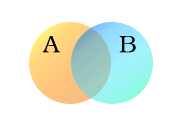Sets A (creatures with two legs) and B (creatures that can fly)

The following example uses two sets, A and B, represented here as coloured circles. The orange circle, set A, represents all living creatures that are two-legged. The blue circle, set B, represents the living creatures that can fly. Each separate type of creature can be imagined as a point somewhere in the diagram. Living creatures that can both fly and have two legs—for example, parrots—are then in both sets, and hence correspond to points in the area where the blue and orange circles overlap. That area contains all such (and only) such living creatures.

Humans and penguins are bipedal, and hence are in the orange circle, but since they cannot fly, they appear in the left part of the orange circle, where it does not overlap with the blue circle. Mosquitoes have six legs, and fly, so the point for mosquitoes is in the part of the blue circle that does not overlap with the orange one. Creatures that are not two-legged and cannot fly (for example, whales and spiders) would all be represented by points outside both circles.

The combined area of sets A and B is called the union of A and B, denoted by A ∪ B. The union in this case contains all living creatures that are either two-legged, or that can fly (or both). The area in both A and B, where the two sets overlap, is called the intersection of A and B, denoted by A ∩ B. For example, the intersection of the two sets is not empty, because there are points that represent creatures that are in both the orange and blue circles.

## Venn diagrams of common operations on sets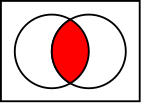Intersection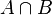$A \cap B$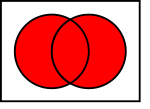Union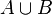$A \cup B$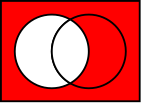Complement of A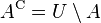$A^{\rm C} = U \setminus A$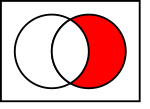Difference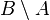$B \setminus A$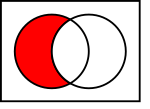Difference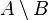$A \setminus B$

In the illustrations below the left circle shows set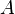$A$ and the right circle shows set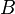$B$. Results of operations are shown as colored areas.

## Images for kidsVenn diagram Facts for Kids. Kiddle Encyclopedia.